# The MI Procedure

### Sensitivity Analysis for the MAR Assumption

Subsections:

Multiple imputation usually assumes that the data are missing at random (MAR). Suppose the data set contains variables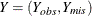, whereare fully observed variables andis a variable that contains missing observations. Also supposeis a response indicator whose element is 0 or 1, depending on whetheris missing or observed. Then the MAR assumption is that the probability that aobservation is missing can depend onbut not on. That is,The MAR assumption cannot be verified, because the missing values are not observed. In clinical trials, for a study that assumes MAR, the sensitivity of inferences to departures from the MAR assumption should be examined, as recommended by the National Research Council (2010, p. 111):

Recommendation 15: Sensitivity analysis should be part of the primary reporting of findings from clinical trials. Examining sensitivity to the assumptions about the missing data mechanism should be a mandatory component of reporting.

If it is plausible that the missing data are not MAR, you can perform sensitivity analysis under the missing not at random (MNAR) assumption. You can generate inferences for various scenarios under MNAR and then examine the results. If the results under MNAR differ from the results under MAR, then the conclusion under MAR is in question.

Based on the factorization of the joint distribution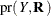, there are two common strategies for sensitivity analysis under MNAR: the pattern-mixture model approach and the selection model approach. The pattern-mixture model approach is implemented in the MI procedure because it is natural and straightforward.

#### Pattern-Mixture Model Approach

In the pattern-mixture model approach (Little 1993; Molenberghs and Kenward 2007, pp. 30, 34–37; National Research Council 2010, pp. 88–89), the joint distribution is factorized as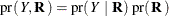This allows for different distributions for missing values and for observed values. For example,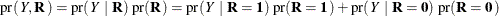which is a mixture of distributions for two different patterns. Here, the "pattern" refers to a group of observations that have the same distribution; the term is not used in the same sense as "missing data pattern."

In the pattern-mixture model approach, the joint distribution is factored asand under the MNAR assumption,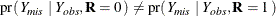It is straightforward to create imputations by using pattern-mixture models. The next three sections provide details for this approach.

#### Selection Model Approach

In the selection model approach (Rubin 1987, p. 207; Little and Rubin 2002, pp. 313–314; Molenberghs and Kenward 2007, p. 30), the joint distribution is factorized as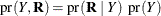where,is the marginal distribution of `Y`, and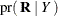is the conditional distribution of the missing mechanism R given `Y`. The term "selection" comes from the specification of R that selects individuals to be observed in the conditional distribution. Both distributions,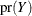and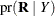, must be specified for the analysis. The MI procedure does not provide this approach.